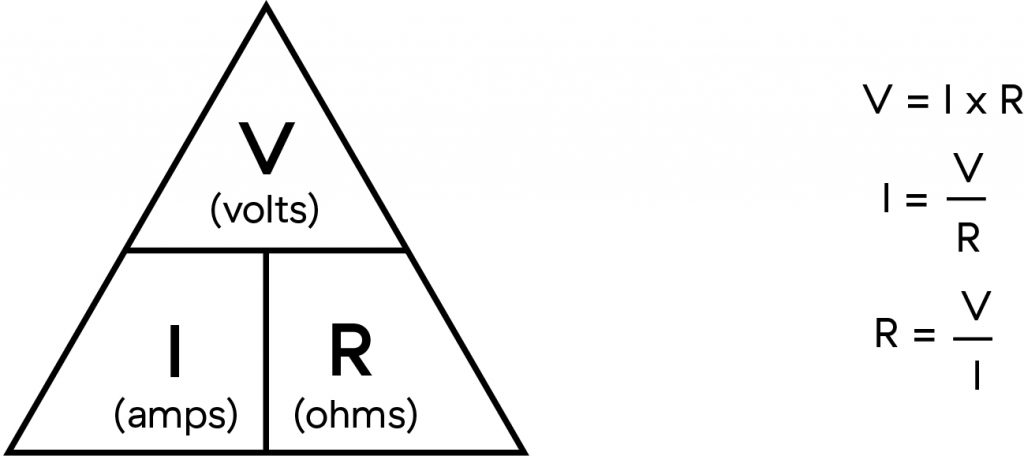We cannot see the electrons that transfer energy in an electrical circuit but we are able to detect their presence using a Voltmeter that indicates the potential difference between two points in a circuit or an Ammeter that indicates the amount of electrons or current flowing in a circuit.

A common analogy used when explaining  electrical principles is to use the flow of water as a way of visualising current flow. If the water flows through a large pipe, there is little resistance to the flow of water, and similarly if an electric current flow through a large copper wire, there is little resistance to the current flow. A smaller size water pipe will restrict water flow and a smaller size copper wire will restrict current flow. A tap can be used to regulate the flow of water and a variable resistor or rheostat can control the flow of electrical current. A remotely controlled valve can regulate the flow of water and a semiconductor junction device such as a transistor or thyristor can regulate the flow of electric current by varying a control signal.

If water is stored in a tank above the ground, gravity is forcing the water down a pipe, ready to flow when a tap or valve is turned. In DC circuits, energy is stored in a battery or made available from a DC Power Supply, and that energy is available when a current can flow through a circuit. The amount of current that flows in a circuit is determined by the energy or potential difference of the battery and the resistance of the circuit elements.

OHMS LAW
Ohms law is the relationship between Voltage, Current and Resistance which is V(volts)  = I (amps) x R (ohms) , so if we have 10 amps flowing in a DC circuit that has a resistance of 10 ohms, then there is a voltage or potential difference of 100 volts across the circuit. This is shown as the ohms law triangle that allows an unknown value to be calculated from the two known values.Useful Info: The Electrodroid app has many calculators including Ohm’s Law, Battery Life, Power, Wire, Resistors in the free version. https://electrodroid.it/electrodroid/ .